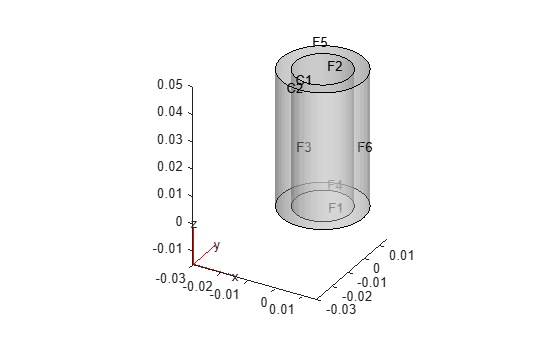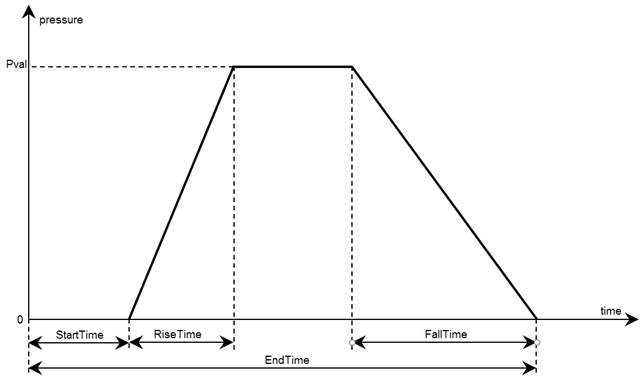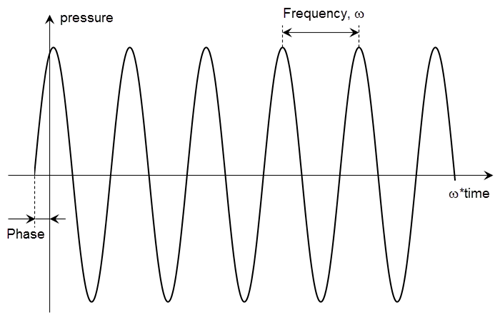Documentation

Specify boundary loads for structural model

## Syntax

``structuralBoundaryLoad(structuralmodel,RegionType,RegionID,'SurfaceTraction',STval,'Pressure',Pval,'TranslationalStiffness',TSval)``
``structuralBoundaryLoad(structuralmodel,'Vertex',VertexID,'Force',Fval)``
``structuralBoundaryLoad(___,'Vectorized','on')``
``structuralBoundaryLoad(___,'Pressure',Pval,Name,Value)``
``structuralBoundaryLoad(structuralmodel,'Vertex',VertexID,'Force',Fval,Name,Value)``
``boundaryLoad = structuralBoundaryLoad(___)``

## Description

example

````structuralBoundaryLoad(structuralmodel,RegionType,RegionID,'SurfaceTraction',STval,'Pressure',Pval,'TranslationalStiffness',TSval)` specifies the surface traction, pressure, and translational stiffness on the boundary of type `RegionType` with `RegionID` ID numbers.Surface traction is determined as distributed normal and tangential forces acting on a boundary, resolved along the global Cartesian coordinate system.Pressure must be specified in the direction that is normal to the boundary. A positive pressure value acts into the boundary (for example, compression). A negative pressure value acts away from the boundary (for example, suction). Translational stiffness is a distributed spring stiffness for each translational direction. Translational stiffness is used to model an elastic foundation.`structuralBoundaryLoad` does not require you to specify all three boundary loads. Depending on your structural analysis problem, you can specify one or more boundary loads by picking the corresponding arguments and omitting others. You can specify translational stiffness for any structural model. To specify pressure or surface traction, `structuralmodel` must be a static, transient, or frequency response model. Structural models for modal analysis cannot have pressure or surface traction.The default boundary load is a stress-free boundary condition.```

example

````structuralBoundaryLoad(structuralmodel,'Vertex',VertexID,'Force',Fval)` specifies concentrated force at a vertex with the `VertexID` number. You can specify force only if `structuralmodel` is a static, transient, or frequency response model. Structural models for modal analysis cannot have concentrated force.```
````structuralBoundaryLoad(___,'Vectorized','on')` uses vectorized function evaluation when you pass a function handle as an argument. If your function handle computes in a vectorized fashion, then using this argument saves time. See Vectorization (MATLAB). For details on this evaluation, see Nonconstant Boundary Conditions.Use this syntax with any of the input arguments from previous syntaxes.```

example

````structuralBoundaryLoad(___,'Pressure',Pval,Name,Value)` lets you specify the form and duration of a nonconstant pressure pulse and harmonic excitation for a transient structural model without creating a function handle. When using this syntax, you must specify the model, region type and region ID, and pressure. Surface traction and translational stiffness are optional arguments. This syntax does not work for static, modal analysis, and frequency response models.```

example

````structuralBoundaryLoad(structuralmodel,'Vertex',VertexID,'Force',Fval,Name,Value)` lets you specify the form and duration of a nonconstant concentrated force and harmonic excitation for a transient structural model without creating a function handle.```
````boundaryLoad = structuralBoundaryLoad(___)` returns the boundary load object.```

## Examples

collapse all

Apply fixed boundaries and traction on two ends of a bimetallic cable.

Create a structural model.

`structuralModel = createpde('structural','static-solid');`

Create nested cylinders to model a bimetallic cable.

`gm = multicylinder([0.01,0.015],0.05);`

Assign the geometry to the structural model and plot the geometry.

```structuralModel.Geometry = gm; pdegplot(structuralModel,'CellLabels','on','FaceLabels','on','FaceAlpha',0.4)```For each metal, specify the Young's modulus and Poisson's ratio.

```structuralProperties(structuralModel,'Cell',1,'YoungsModulus',110E9, ... 'PoissonsRatio',0.28); structuralProperties(structuralModel,'Cell',2,'YoungsModulus',210E9, ... 'PoissonsRatio',0.3);```

Specify that faces 1 and 4 are fixed boundaries.

`structuralBC(structuralModel,'Face',[1,4],'Constraint','fixed')`
```ans = StructuralBC with properties: RegionType: 'Face' RegionID: [1 4] Vectorized: 'off' Boundary Constraints and Enforced Displacements Displacement: [] XDisplacement: [] YDisplacement: [] ZDisplacement: [] Constraint: "fixed" Radius: [] Reference: [] Boundary Loads Force: [] SurfaceTraction: [] Pressure: [] TranslationalStiffness: [] ```

Specify the surface traction for faces 2 and 5.

`structuralBoundaryLoad(structuralModel,'Face',[2,5],'SurfaceTraction',[0;0;100])`
```ans = StructuralBC with properties: RegionType: 'Face' RegionID: [2 5] Vectorized: 'off' Boundary Constraints and Enforced Displacements Displacement: [] XDisplacement: [] YDisplacement: [] ZDisplacement: [] Constraint: [] Radius: [] Reference: [] Boundary Loads Force: [] SurfaceTraction: [3x1 double] Pressure: [] TranslationalStiffness: [] ```

Create a structural model.

`structuralModel = createpde('structural','static-solid');`

Create a block geometry.

`gm = multicuboid(20,10,5);`

Assign the geometry to the structural model and plot the geometry.

```structuralModel.Geometry = gm; pdegplot(structuralModel,'FaceLabels','on','FaceAlpha',0.5)```Specify the Young's modulus and Poisson's ratio.

```structuralProperties(structuralModel,'YoungsModulus',30, ... 'PoissonsRatio',0.3);```

The bottom face of the block rests on an elastic foundation (a spring). To model this foundation, specify the translational stiffness.

`structuralBoundaryLoad(structuralModel,'Face',1,'TranslationalStiffness',[0;0;30])`
```ans = StructuralBC with properties: RegionType: 'Face' RegionID: 1 Vectorized: 'off' Boundary Constraints and Enforced Displacements Displacement: [] XDisplacement: [] YDisplacement: [] ZDisplacement: [] Constraint: [] Radius: [] Reference: [] Boundary Loads Force: [] SurfaceTraction: [] Pressure: [] TranslationalStiffness: [3x1 double] ```

Specify a force value at a vertex of a geometry.

Create a structural model for static analysis of a solid (3-D) problem.

`model = createpde('structural','static-solid');`

Create the geometry, which consists of two cuboids stacked on top of each other.

`gm = multicuboid(0.2,0.01,[0.01 0.01],'Zoffset',[0 0.01]);`

Include the geometry in the structural model.

`model.Geometry = gm;`

Plot the geometry and display the face labels. Rotate the geometry so that you can see the face labels on the left side.

```figure pdegplot(model,'FaceLabels','on'); view([-67 5])```Plot the geometry and display the vertex labels. Rotate the geometry so that you can see the vertex labels on the right side.

```figure pdegplot(model,'VertexLabels','on','FaceAlpha',0.5) xlim([-0.01 0.1]) zlim([-0.01 0.02]) view([60 5])```Specify the Young's modulus, Poisson's ratio, and mass density of the material.

`structuralProperties(model,'YoungsModulus',201E9,'PoissonsRatio',0.3);`

Specify that faces 5 and 10 are fixed boundaries.

`structuralBC(model,'Face',[5 10],'Constraint','fixed');`

Specify the concentrated force at vertex 6.

`structuralBoundaryLoad(model,'Vertex',6,'Force',[0;10^4;0])`
```ans = StructuralBC with properties: RegionType: 'Vertex' RegionID: 6 Vectorized: 'off' Boundary Constraints and Enforced Displacements Displacement: [] XDisplacement: [] YDisplacement: [] ZDisplacement: [] Constraint: [] Radius: [] Reference: [] Boundary Loads Force: [3x1 double] SurfaceTraction: [] Pressure: [] TranslationalStiffness: [] ```

Use a function handle to specify a frequency-dependent pressure for a frequency response model.

Create a frequency response model for a 3-D problem.

`fmodel = createpde('structural','frequency-solid');`

Import and plot the geometry.

```importGeometry(fmodel,'TuningFork.stl'); figure pdegplot(fmodel,'FaceLabels','on')```Specify the pressure loading on a tine (face 11) as a short rectangular pressure pulse. In the frequency domain, this pressure pulse is a unit load uniformly distributed across all frequencies.

`structuralBoundaryLoad(fmodel,'Face',11,'Pressure',1);`

Now specify a frequency-dependent pressure load, for example, $\mathit{p}={\mathit{e}}^{-{\left(\omega -1000\right)}^{2}/100000}$.

```pLoad = @(location,state) exp(-(state.frequency-1E3).^2/1E5); structuralBoundaryLoad(fmodel,'Face',12,'Pressure',pLoad);```

Use a function handle to specify a harmonically varying pressure at the center of a thin 3-D plate.

Create a transient dynamic model for a 3-D problem.

`structuralmodel = createpde('structural','transient-solid');`

Create a geometry consisting of a thin 3-D plate with a small plate at the center. Include the geometry in the model and plot it.

```gm = multicuboid([5,0.05],[5,0.05],0.01); structuralmodel.Geometry = gm; pdegplot(structuralmodel,'FaceLabels','on','FaceAlpha',0.5)```Zoom in to see the face labels on the small plate at the center.

```figure pdegplot(structuralmodel,'FaceLabels','on','FaceAlpha',0.25) axis([-0.2 0.2 -0.2 0.2 -0.1 0.1])```Specify the Young's modulus, Poisson's ratio, and mass density of the material.

```structuralProperties(structuralmodel,'YoungsModulus',210E9,... 'PoissonsRatio',0.3,... 'MassDensity',7800);```

Specify that all faces on the periphery of the thin 3-D plate are fixed boundaries.

`structuralBC(structuralmodel,'Constraint','fixed','Face',5:8);`

Apply a harmonically varying pressure load on the small face at the center of the plate.

```plungerLoad = @(location,state)5E7.*sin(25.*state.time); structuralBoundaryLoad(structuralmodel,'Face',12,'Pressure',plungerLoad)```
```ans = StructuralBC with properties: RegionType: 'Face' RegionID: 12 Vectorized: 'off' Boundary Constraints and Enforced Displacements Displacement: [] XDisplacement: [] YDisplacement: [] ZDisplacement: [] Constraint: [] Radius: [] Reference: [] Boundary Loads Force: [] SurfaceTraction: [] Pressure: @(location,state)5E7.*sin(25.*state.time) TranslationalStiffness: [] Time Variation of Force, Pressure, or Enforced Displacement StartTime: [] EndTime: [] RiseTime: [] FallTime: [] Sinusoidal Variation of Force, Pressure, or Enforced Displacement Frequency: [] Phase: [] ```

Specify a harmonically varying pressure at the center of a thin 3-D plate by specifying its frequency.

Create a transient dynamic model for a 3-D problem.

`structuralmodel = createpde('structural','transient-solid');`

Create a geometry consisting of a thin 3-D plate with a small plate at the center. Include the geometry in the model and plot it.

```gm = multicuboid([5,0.05],[5,0.05],0.01); structuralmodel.Geometry=gm; pdegplot(structuralmodel,'FaceLabels','on','FaceAlpha',0.5)```Zoom in to see the face labels on the small plate at the center.

```figure pdegplot(structuralmodel,'FaceLabels','on','FaceAlpha',0.25) axis([-0.2 0.2 -0.2 0.2 -0.1 0.1])```Specify the Young's modulus, Poisson's ratio, and mass density of the material.

```structuralProperties(structuralmodel,'YoungsModulus',210E9,... 'PoissonsRatio',0.3,... 'MassDensity',7800);```

Specify that all faces on the periphery of the thin 3-D plate are fixed boundaries.

`structuralBC(structuralmodel,'Constraint','fixed','Face',5:8);`

Apply a harmonically varying pressure load on the small face at the center of the plate.

`structuralBoundaryLoad(structuralmodel,'Face',12,'Pressure',5E7,'Frequency',25)`
```ans = StructuralBC with properties: RegionType: 'Face' RegionID: 12 Vectorized: 'off' Boundary Constraints and Enforced Displacements Displacement: [] XDisplacement: [] YDisplacement: [] ZDisplacement: [] Constraint: [] Radius: [] Reference: [] Boundary Loads Force: [] SurfaceTraction: [] Pressure: 50000000 TranslationalStiffness: [] Time Variation of Force, Pressure, or Enforced Displacement StartTime: [] EndTime: [] RiseTime: [] FallTime: [] Sinusoidal Variation of Force, Pressure, or Enforced Displacement Frequency: 25 Phase: [] ```

Create a transient structural model.

`structuralModel = createpde('structural','transient-solid');`

Import and plot the geometry.

```importGeometry(structuralModel,'BracketWithHole.stl'); pdegplot(structuralModel,'FaceLabels','on') view(-20,10)```Specify the Young's modulus and Poisson's ratio.

```structuralProperties(structuralModel,'YoungsModulus',200e9, ... 'PoissonsRatio',0.3,... 'MassDensity',7800);```

Specify that face 4 is a fixed boundary.

`structuralBC(structuralModel,'Face',4,'Constraint','fixed');`

Apply a rectangular pressure pulse on face 7 in the direction normal to the face.

```structuralBoundaryLoad(structuralModel,'Face',7,'Pressure',10^5,... 'StartTime',0.1,'EndTime',0.5)```
```ans = StructuralBC with properties: RegionType: 'Face' RegionID: 7 Vectorized: 'off' Boundary Constraints and Enforced Displacements Displacement: [] XDisplacement: [] YDisplacement: [] ZDisplacement: [] Constraint: [] Radius: [] Reference: [] Boundary Loads Force: [] SurfaceTraction: [] Pressure: 100000 TranslationalStiffness: [] Time Variation of Force, Pressure, or Enforced Displacement StartTime: 0.1000 EndTime: 0.5000 RiseTime: [] FallTime: [] Sinusoidal Variation of Force, Pressure, or Enforced Displacement Frequency: [] Phase: [] ```

Specify a short concentrated force pulse at a vertex of a geometry.

Create a structural model for static analysis of a solid (3-D) problem.

`structuralmodel = createpde('structural','transient-solid');`

Create the geometry, which consists of two cuboids stacked on top of each other.

`gm = multicuboid(0.2,0.01,[0.01 0.01],'Zoffset',[0 0.01]);`

Include the geometry in the structural model.

`structuralmodel.Geometry = gm;`

Plot the geometry and display the face labels. Rotate the geometry so that you can see the face labels on the left side.

```figure pdegplot(structuralmodel,'FaceLabels','on'); view([-67 5])```Plot the geometry and display the vertex labels. Rotate the geometry so that you can see the vertex labels on the right side.

```figure pdegplot(structuralmodel,'VertexLabels','on','FaceAlpha',0.5) xlim([-0.01 0.1]) zlim([-0.01 0.02]) view([60 5])```Specify the Young's modulus, Poisson's ratio, and mass density of the material.

```structuralProperties(structuralmodel,'YoungsModulus',201E9, ... 'PoissonsRatio',0.3, ... 'MassDensity',7800);```

Specify that faces 5 and 10 are fixed boundaries.

`structuralBC(structuralmodel,'Face',[5 10],'Constraint','fixed');`

Specify a short concentrated force pulse at vertex 6.

```structuralBoundaryLoad(structuralmodel,'Vertex',6,'Force',[0;1000;0], ... 'StartTime',1,'EndTime',1.05)```
```ans = StructuralBC with properties: RegionType: 'Vertex' RegionID: 6 Vectorized: 'off' Boundary Constraints and Enforced Displacements Displacement: [] XDisplacement: [] YDisplacement: [] ZDisplacement: [] Constraint: [] Radius: [] Reference: [] Boundary Loads Force: [3×1 double] SurfaceTraction: [] Pressure: [] TranslationalStiffness: [] Time Variation of Force, Pressure, or Enforced Displacement StartTime: 1 EndTime: 1.0500 RiseTime: [] FallTime: [] Sinusoidal Variation of Force, Pressure, or Enforced Displacement Frequency: [] Phase: [] ```

Specify zero initial displacement and velocity.

`structuralIC(structuralmodel,'Displacement',[0;0;0],'Velocity',[0;0;0]) `
```ans = GeometricStructuralICs with properties: RegionType: 'Cell' RegionID: [1 2] InitialDisplacement: [3×1 double] InitialVelocity: [3×1 double] ```

Generate a fine mesh.

`generateMesh(structuralmodel,'Hmax',0.02);`

Because the load is zero for the initial time span and is applied for only a short time, solve the model for two time spans. Use the first time span to find the solution before the force pulse.

`structuralresults1 = solve(structuralmodel,0:1E-2:1); `

Use the second time span to find the solution during and after the force pulse.

`structuralIC(structuralmodel,structuralresults1)`
```ans = NodalStructuralICs with properties: InitialDisplacement: [511×3 double] InitialVelocity: [511×3 double] ```
`structuralresults2 = solve(structuralmodel,[1.001:0.001:1.01 1.02:1E-2:2]);`

Plot the displacement value at the node corresponding to vertex 6, where you applied the concentrated force pulse.

```loadedNd = findNodes(structuralmodel.Mesh,'region','Vertex',6); plot(structuralresults2.SolutionTimes,structuralresults2.Displacement.uy(loadedNd,:))```## Input Arguments

collapse all

Structural model, specified as a `StructuralModel` object. The model contains the geometry, mesh, structural properties of the material, body loads, boundary loads, and boundary conditions.

Example: ```structuralmodel = createpde('structural','transient-solid')```

Geometric region type, specified as `'Edge'` for a 2-D model or `'Face'` for a 3-D model.

Example: `structuralBoundaryLoad(structuralmodel,'Face',[2,5],'SurfaceTraction',[0,0,100])`

Data Types: `char` | `string`

Geometric region ID, specified as a positive integer or vector of positive integers. Find the region IDs by using `pdegplot`.

Example: `structuralBoundaryLoad(structuralmodel,'Face',[2,5],'SurfaceTraction',[0,0,100])`

Data Types: `double`

Vertex ID, specified as a positive integer or vector of positive integers. Find the vertex IDs using `pdegplot`.

Example: `structuralBoundaryLoad(structuralmodel,'Vertex',6,'Force',[0;10^4;0])`

Data Types: `double`

Distributed normal and tangential forces on the boundary, resolved along the global Cartesian coordinate system, specified as a numeric vector or function handle. A numeric vector must contain two elements for a 2-D model and three elements for a 3-D model. The function must return a two-row matrix for a 2-D model and a three-row matrix for a 3-D model. Each column of the matrix must correspond to the surface traction vector at the boundary coordinates provided by the solver. In case of a transient structural model, `STval` also can be a function of time.

Example: `structuralBoundaryLoad(structuralmodel,'Face',[2,5],'SurfaceTraction',[0;0;100])`

Data Types: `double` | `function_handle`

Pressure normal to the boundary, specified as a number or function handle. The function must return a row vector where each column corresponds to the value of pressure at the boundary coordinates provided by the solver. A positive-value pressure acts into the boundary (for example, compression), while a negative-value pressure acts away from the boundary (for example, suction).

In case of a transient structural model, `Pval` also can be a function of time. In case of a frequency response structural model, `Pval` can be a function of frequency (when specified as a function handle) or a constant pressure with the same magnitude for a broad frequency spectrum.

Example: `structuralBoundaryLoad(structuralmodel,'Face',[2,5],'Pressure',10^5)`

Data Types: `double` | `function_handle`

Distributed spring stiffness for each translational direction used to model elastic foundation, specified as a numeric vector or function handle. A numeric vector must contain two elements for a 2-D model and three elements for a 3-D model. The custom function must return a two-row matrix for a 2-D model and a three-row matrix for a 3-D model. Each column of this matrix corresponds to the stiffness vector at the boundary coordinates provided by the solver. In case of a transient structural model, `TSval` also can be a function of time.

Example: `structuralBoundaryLoad(structuralmodel,'Edge',[2,5],'TranslationalStiffness',[0;5500])`

Data Types: `double` | `function_handle`

Concentrated force at a vertex, specified as a numeric vector or function handle.

Example: `structuralBoundaryLoad(structuralmodel,'Vertex',5,'Force',[0;0;10])`

Data Types: `double` | `function_handle`

### Name-Value Pair Arguments

Example: `structuralBoundaryLoad(structuralmodel,'Face',[2,5],'Pressure',10^5,'RiseTime',0.5,'FallTime',0.5,'EndTime',3)`

Use one or more of the name-value pair arguments to specify the form and duration of the pressure or concentrated force pulse and harmonic excitation for a transient structural model only. Specify the pressure or force value using the `Pval` or `Fval` argument, respectively.

You can model rectangular, triangular, and trapezoidal pressure or concentrated force pulses. If the start time is 0, you can omit specifying it.

• For a rectangular pulse, specify the start and end times.

• For a triangular pulse, specify the start time and any two of the following times: rise time, fall time, and end time. You also can specify all three times, but they must be consistent.

• For a trapezoidal pulse, specify all four times.You can model a harmonic pressure or concentrated force load by specifying its frequency and initial phase. If the initial phase is 0, you can omit specifying it.#### Rectangular, Triangular, or Trapezoidal Pulse

collapse all

Start time for pressure or concentrated force load, specified as a nonnegative number. Specify this argument only for transient structural models.

Example: `structuralBoundaryLoad(structuralmodel,'Face',[2,5],'Pressure',10^5,'StartTime',1,'EndTime',3)`

Data Types: `double`

End time for pressure or concentrated force load, specified as a nonnegative number equal or greater than the start time value. Specify this argument only for transient structural models.

Example: `structuralBoundaryLoad(structuralmodel,'Face',[2,5],'Pressure',10^5,'StartTime',1,'EndTime',3)`

Data Types: `double`

Rise time for pressure or concentrated force load, specified as a nonnegative number. Specify this argument only for transient structural models.

Example: `structuralBoundaryLoad(structuralmodel,'Face',[2,5],'Pressure',10^5,'RiseTime',0.5,'FallTime',0.5,'EndTime',3)`

Data Types: `double`

Fall time for pressure or concentrated force load, specified as a nonnegative number. Specify this argument only for transient structural models.

Example: `structuralBoundaryLoad(structuralmodel,'Face',[2,5],'Pressure',10^5,'RiseTime',0.5,'FallTime',0.5,'EndTime',3)`

Data Types: `double`

#### Harmonic Pressure or Force

collapse all

Frequency of sinusoidal pressure or concentrated force, specified as a positive number, in radians per unit of time. Specify this argument only for transient structural models.

Example: `structuralBoundaryLoad(structuralmodel,'Face',[2,5],'Pressure',10^5,'Frequency',25)`

Data Types: `double`

Phase of sinusoidal pressure or concentrated force, specified as a nonnegative number, in radians. Specify this argument only for transient structural models.

Example: `structuralBoundaryLoad(structuralmodel,'Face',[2,5],'Pressure',10^5,'Frequency',25,'Phase',pi/6)`

Data Types: `double`

## Output Arguments

collapse all

Handle to boundary load, returned as a `StructuralBC` object.